Geometry > Curves and ShapesShowing results 1 to 10 of 19, on page 1 of 2
 DESCRIPTION EQUATION Distance Between Two Points (2-D)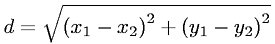Distance Between Two Points (3-D)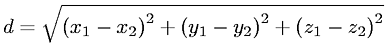Eccentricity of a HyperbolaEccentricity of an EllipseEquation of a Circle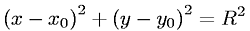Equation of a Hyperbola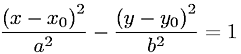Equation of a Hyperbolic Paraboloid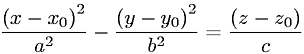Equation of a Hyperboloid of One Sheet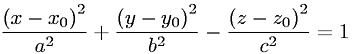Equation of a Hyperboloid of Two Sheets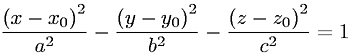Equation of a Line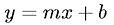Go to page: 1  2